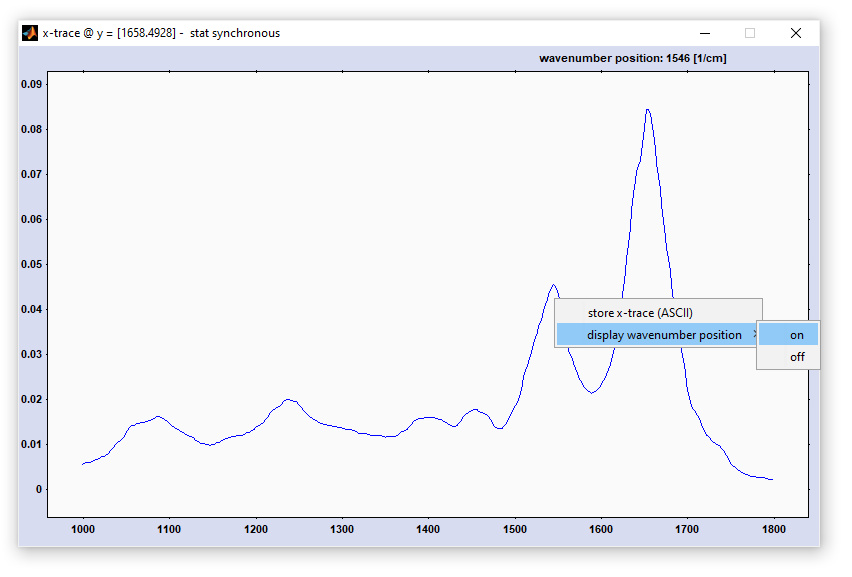## CytoSpec - an APPLICATION FOR HYPERSPECTRAL IMAGING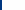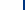File Pulldown Menu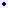LoadSaveSave MatlabImport ASCIIImport BinaryExportDeleteClearPlotCustomizeBatch Multiple FilesExit   Spectral PreprocessingCalculation of Derivative SpectraNode AttenuationNormalizationCut SpectraInterpolate SpectraSmooth SpectraABS ↔ TR ConversionSubtractionSpectral Quality TestsBaseline CorrectionWater Vapor CorrectionNoise ReductionCosmic Spike RemovalFourier Self-DeconvolutionBatch Preprocessing Spatial PreprocessingCropInterpolate/BinningReplace NaNsFilter ImagesEdge Preserving Denoising3D-FSD   Univariate ImagingChemical ImagingChemical MovieFrequency ImagingFWHM Imaging  Multivariate ImagingHCA ImagingKMC ImagingFCM Cluster ImagingPCA ImagingVCA Imagingn-findr ImagingANN ImagingSynthon ImagingImaging with Distance ValuesMCR-ALS imagingCreate Composite ImagesHCA of Chemical Images ToolsDisplay SpectraSet Display LimitsGrid On/OffAdapt ColormapsCapture ImagesExport Image DataImage StatisticsDisplay Large Images2D-COSDefine ROIDisplay ColorbarSwap Data BlocksRotate HSIFlip HSI   File InformationShow HistoryShow Instrument ParametersShow Measurement ParametersShow Additional ParametersEdit ParametersAboutUsing the Help Function  Glossary

## Two-Dimensional Correlation Spectroscopy (2D-COS)

#### Two-dimensional correlation spectroscopy (2D-COS), or two-dimensional correlation analysis is known as a set of mathematical techniques useful to study changes in dynamic spectra. Dynamic spectra are often represented by spectra series obtained from a sample that was subjected to an external perturbation. The implementation of 2D-COS in the CytoSpec software aims to enable the analysis of spatially resolved image (HSI) data. For this purpose a variety of methods and procedures are available, which are explained in detail in this section of the online help. Application examples of the 2D-COS technique can be found in two scientific publications, see links at the end of this section ('Reference to the literature'). The 2D-COS analysis technique has been initially developed byIsao Noda in the 1980s. Related web links:Two-dimensional correlation analysis (Wikipedia)Mat2dcorr - A Matlab Toolbox for 2D-COS. This Wiki provides a detailled description of the free 2D-COS toolbox for Matlab and allows downloding the corresponding source code. Note that a modified version of the toolbox' source code has been used to compile CytoSpec's 2D-COS function. Main concepts of 2D correlation spectroscopy: Basic principles of generalized 2D correlation spectroscopy are outlined in the following publication series:I. Noda.Two-Dimensional Infrared (2D IR) Spectroscopy: Theory and Applications,   1990 Appl. Spectrosc. 44(4): 550-561I. Noda.Generalized Two- Dimensional Correlation Method Applicable to Infrared, Raman, and other Types of Spectroscopy,   1993 Appl. Spectrosc. 47(9): 1329-1336I. Noda.Determination of Two-Dimensional Correlation Spectra Using the Hilbert Transform,   2000 Appl. Spectrosc. 54(7): 994-999   To start a 2D-COS analysis select '2D-COS' from the 'Tools' menu bar. This will open two different figures: A figure entitled '2D correlation analysis ... ' which shows the 2D correlation spectrum and mean spectra obtained from the x- and y-spectra data The '2D control' figure, see description and screenshots below Two-Dimensional Correlation Spectroscopy (2D-COS) - the 2D correlation spectrum windowOption 1.)
This options obtains x, or y-data directly from the CytoSpec workspace. Select 'Get Data' → 'Get x-data' → 'from original/ preprocessed /derivative / deconvolution data' to get the x-data. To obtain the respective y-data select 'Get Data' → 'y-data' → 'etc.'. The 'Get Data' option is available from the menu bar of the window entitled '2D correlation analysis ... ' (see window above).
Note that option 1 does not allow performing heterospectral 2D correlation analysis.

Option 2.)
Load data in the Matlab imaging format: Select 'Load Data' → 'Matlab imaging format' → 'x-data' from the menu bar of the 2D-COS window to load x-data in the Matlab imaging data format. Select 'Load Data' → 'Matlab imaging format' → 'y-data' to load the respective y-data in the Matlab imaging data format. Matlab imaging data files may contain up to four different types of hyperspectral imaging data cubes: original (unprocessed data), pre-processed, derivative and so-called deconvolution data cubes (the latter 3 types of data must be derived from the original spectral data). For a detailed description of the Matlab imaging data format see http://www.cytospec.com/file.php#FileSaveMatlab

Option 3.)
Load data in the Matlab trace format: Select 'Load Data' → 'Matlab trace format' → 'x-data' to load x-data in the Matlab trace data format. Select 'Load Data' → 'Matlab trace format' → 'y-data' to load the respective y-data in the Matlab trace data format.
For a description of the Matlab trace format see the section below.

Format of the example file 'linescandata.mat' (Matlab trace format)
Matlab trace format files contain spectra series in a 2D data format where the first dimension is the spectral dimension and the second dimension represents the perturbing variable, i.e. time, pressure, temperature, spatial dimension, etc.

Matlab trace files contain a structure array with the following fields:

1. spc - this field contains the spectral data, a 2D array of double precision floating point values (float32). Columns indicate individual spectra of absorbance, intensity, transmittance, etc. values. The column length indicates the number of data points per spectrum. The number of columns is equal to the number of spectra of the spectra series.
2. wav - A vector of float32 values, the 'wavenumber' vector, or more general the vector of y-values (frequencies, wavenumbers, Raman shifts or alternative variables). The length of 'wav' must equal the number of data points per spectrum, i.e. size(wav,1) == size(spec,1). Equidistancy of the 'wav' vector is not a requirement.
3. tos - A character vector of variable length which indicates the type of spectra. Examples: 'Transmission', 'Fluorescence', 'Raman', etc.
4. war - A vector of float32 values, which denote the perturbing variable of the experiment: temperature, time, voltage. The length of 'war' must be equal to size(spc,2)
5. vst - A character vector of variable length indicating the type of the perturbing variable. Examples of vst: 'Temperature', 'Time', 'Voltage', or 'Spatial variable'

Options of the 2D-COS interactive user dialog box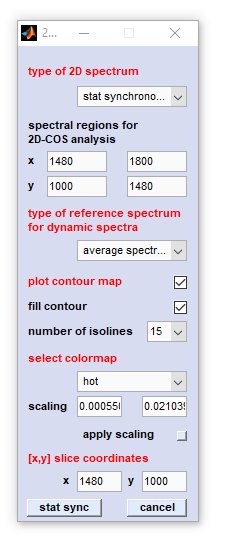Screenshot of the dialog box for two-dimensional correlation spectroscopy (2D-COS) type of 2D spectrum: defines the type of the 2D-COS analysis, for details see below   spectral regions for 2D-COS analyses: allows to define the [x,y] spectral ranges for 2D-COS   type of reference spectrum: defines the type of reference spectrum to obtain the dynamic spectrum. Valid options are 'no reference' (spectrum), 'average spectrum', (default) 'first spectrum' and 'last spectrum'.   plot contour map: plots a contour map (instead of an interpolated surface map where the color is proportional to the 2D-COS functional values).   fill contour: creates a filled contour map   number of isolines: defines the number of isolines in contour / filled contour maps   select colormap: the type of color maps used to plot surface maps, or to plot the isolines in contour maps   scaling: permits to modify manually the color map by entering the minimal and maximal z-values into the appropriate edit boxes.   apply scaling: scaling values are immediately applied to the colormap when this button was pressed   [x,y] slice coordinates: settings required to plot 1D correlation slices, or to create [x,y] feature plots

Options of the popupmenu 'type of 2D spectrum'
1. Pearson scaling: the synchronous 2D spectrum with Pearson, or unit variance scaling. Pearson scaling is also used in statistical total correlation spectroscopy [STOCSY] and statistical heterospectroscopy [SHY])
2. Pareto scale 0.75: the synchronous 2D spectrum with Pareto scaling. The parameter α equals 0.75
3. Pareto scale 0.50: the synchronous 2D spectrum with Pareto scaling. The parameter α equals 0.50 (Pareto scaling in the strict sense)
4. Pareto scale 0.25: the synchronous 2D spectrum with Pareto scaling. The parameter α equals 0.25
5. stat synchronous: the classical (statistical) synchronous 2D (covariance) spectrum
6. stat asynchronous: the classical (statistical) asynchronous 2D spectrum
7. disrelation: allows to calculate the absolute of the 2D disrelation spectrum
8. fft synchronous: alternative implementation to obtain the synchronous 2D correlation spectrum by means of the fast Fourier-transformation approach
9. fft asynchronous: alternative implementation to obtain the asynchronous 2D correlation spectrum by means of the fast Fourier-transformation approach